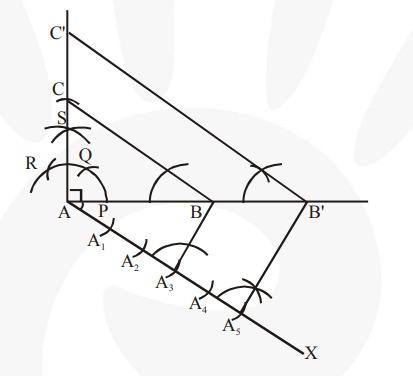# Draw a right triangle in which the sides (other than hypotenuse) are of lengths 4 cm and 3 cm.Question:

Draw a right triangle in which the sides (other than hypotenuse) are of lengths 4 cm and 3 cm.Then construct another triangle whose sides are $\frac{\mathbf{5}}{\mathbf{3}}$ times the corresponding sides of the given triangle.

Solution:

Steps of construction :

1. Draw a line segment AB = 4 cm. Draw a ray SA making 90° with it.

2. Draw an arc of 3 cm radius while taking A as its centre to intersect SA at C. Join BC.

$\triangle \mathrm{ABC}$ is the required triangle.

3. Draw a ray AX making an acute angle with AB, opposite to vertex C.

4. Mark 5 points (as 5 is greater in 5 and 3$), A_{1}, A_{2}, A_{3}, A_{4}, A_{5}$, on line segment $A X$ such that $\mathrm{AA}_{1}=\mathrm{A}_{1} \mathrm{~A}_{2}=\mathrm{A}_{2} \mathrm{~A}_{3}=\mathrm{A}_{3} \mathrm{~A}_{4}=\mathrm{A}_{4} \mathrm{~A}_{5} .$

5. Join $A_{3} B$. Draw a line through $A_{5}$ parallel to $A_{3} B$ intersecting extended line segment $A B$ at B'.

6. Through $\mathrm{B}^{\prime}$, draw a line parallel to $\mathrm{BC}$ intersecting extended line segment $\mathrm{AC}$ at $\mathrm{C}^{\prime}$.

$\triangle \mathrm{AB}^{\prime} \mathrm{C}^{\prime}$ is the required triangle.